Courses

# Short Answer Type Questions- Linear Equations in Two Variables Class 9 Notes | EduRev

## Mathematics (Maths) Class 9

Created by: Full Circle

## Class 9 : Short Answer Type Questions- Linear Equations in Two Variables Class 9 Notes | EduRev

The document Short Answer Type Questions- Linear Equations in Two Variables Class 9 Notes | EduRev is a part of the Class 9 Course Mathematics (Maths) Class 9.
All you need of Class 9 at this link: Class 9

Question 1. Is (3, 2) a solution of x + y = 6?
Solution: (3, 2) means x = 3 and y = 2
∴ Substituting x = 3 and y = 2 in x + y = 6,
we have 3 + 2 = 6
⇒ 5 = 6 which is not correct Since
L.H.S. ≠ R.H.S.
∴ (3, 2) is not a solution of x + y = 6.

Question 2. Is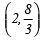a solution of 2x + 3y = 12?
Solution: The given equation is 2x + 3y = 12  ...(1)

Here Solution =⇒ x = 2 and y = (8/3)
Substituting x = 2 and y =(8/3)  in (1), we get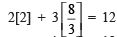⇒ 4 + 8 = 12
⇒ 12 = 12
∵ L.H.S. = R.H.Sis a solution of 2x + 3y = 12.

Question 3. (i) Write the equation of x-axis.
(ii) Write the equation of y-axis.
Solution: (i) Since, the y-coordinate is zero at every point on the x-axis.
∴ The equation of x-axis is y = 0.
(ii) Since, the x-coordinate is zero at every point on the y-axis.
∴ The equation of y-axis is x = 0.

Question 4. Express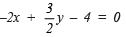in the form of ax + by + c = 0 and write the value of a, b and c.
Solution: We have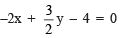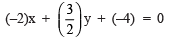...(1)

Comparing (1) with ax + by + c = 0, we have
a = –2, b = (3/2) and c = –4.

Question 5. Express 2x = 5 in the form ax + by + c = 0 and find the value of a, b and c.
Solution: 2x = 5 can be written as 2x – 5 = 0
⇒ 2x + (0)y – 5 = 0
⇒ 2x + (0)y + (–5) = 0                                ...(1)
Comparing (1) with ax + by + c = 0, we get
a = 2,  b = 0 and  c = –5.

Question 6. Write two solutions of 3x + y = 8.
Solution: We have 3x + y = 8
For x = 0, we have 3(0) + y = 8
⇒ 0 x y= 8 ⇒ y = 8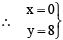.e. (0, 8) is a solution.
For x = 1, we have 3(1) + y = 8
⇒ 3 + y = 8
⇒ y = 8 – 3 = 5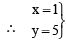i.e. (1, 5) is another solution.

Question 7. If x = –1 and y = 2 is a solution of kx + 3y = 7, find the value k.
Solution: We have kx + 3y = 7                          ...(1)
∴ Putting x = –1 and y = 2 in (1), we get
k(–1) + 3(2) = 7
⇒ –k + 6 = 7
⇒ –k = 7 – 6 = 1
⇒ k= –1
Thus, the required value of k = –1.

Question 8. Show that x = 2 and y = 1 satisfy the linear equation 2x + 3y = 7.
Solution: We have 2x + 3y = 7                       ...(1)
Since, x = 2 and y = 1 satisfy the equation (1).
∴ Substituting x = 2 and y = 1 in (1), we get
L.H.S. = 2(2) + 3(1) = 4 + 3 = 7
= R.H.S.
Since, L.H.S. = R.H.S.
∴ x = 2 and y = 1 satisfy the given equation.

Question 9. Write four solutions of 2x + 3y = 8.
Solution: We have 2x + 3y = 8   ...(1)
Let us assume x = 0.
∴ Substituting x = 0 in (1), we get
2(0) + 3y = 8
⇒ 0 + 3y = 8
⇒ y= (8/3)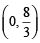is a solution of (1)

Again assume y = 0.
∴ From (1), we have
2x + 3(0) = 8
⇒ 2x = 8
⇒   x= (8/2)  = 4

∴ (4, 0) is a solution of (1).
Again assume x = 1.
∴ From (1), we have
2(1) + 3y = 8
⇒ 3y = 8 – 2 = 6
⇒ y = (6/3) = 2
∴ (1, 2) is a solution of (1).
Again assume x = 2.
∴ From (1), we have
2(2) + 3y = 8
⇒ 4 + 3y = 8
⇒ 3y = 8 – 4 = 4
⇒ y = (4/3)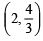is a solution of (1).

∴ The required four solutions are: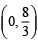,  (4, 0), (1, 2) andQuestion 10. Draw the graph of the equation 2x – 3y = 12. At what points, the graph of the equation cuts the x-axis and the y-axis?
Solution: We have 2x – 3y = 12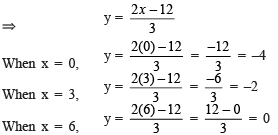∴ We get the following table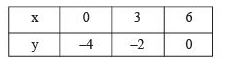Plotting the ordered pairs (0, –4), (3, –2) and (6, 0) and joining them, we get a straight line PQ.
Thus, PQ is the graph of 2x – 3y = 12.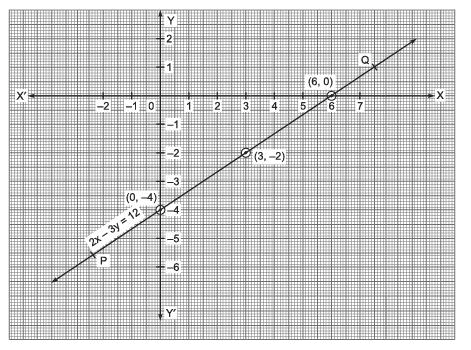From the graph, we see that, it (in line PQ) cuts the x-axis at the point (6, 0) and the y-axis at the point (0, –4).

Question 11. Draw the graph of 9x – 5y + 160 = 0. From the graph find the value of y when x = 5.
Solution: We have: 9x – 5y + 160 = 0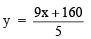When x = 0,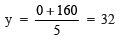When x = –10,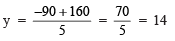When x = –15,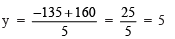We get the table: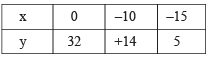Plotting (0, 32), (–10, +14) and (–15, 5) on the graph paper and joining them, we get a straight line.
From the graph, we find for x = 5, the value of y = 41.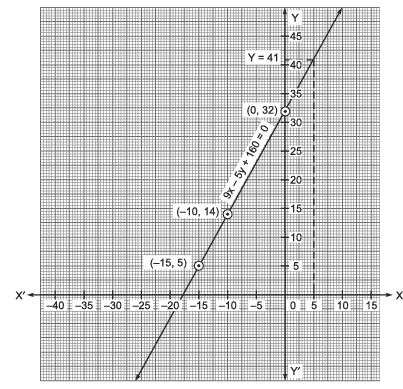Question 12. From the following graph, find four solutions of the equation representing the line AB.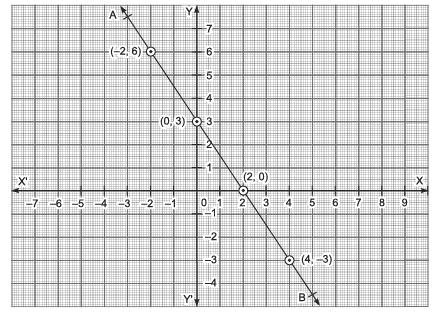Solution: From the graph, we find that the points (2, 0), (0, 3), (4, –3) and (–2, 6) lie on AB.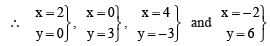are four solutions of the equation representing the straight line AB.

,

,

,

,

,

,

,

,

,

,

,

,

,

,

,

,

,

,

,

,

,

;